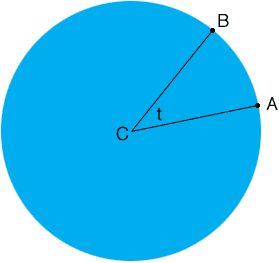Quandaries and Queries Hi, my name is Gretel and I'm a 10th grade student and I'm having a problem with a question for my honors trigonometry class.   My Question is:     Assuming the the earth is a sphere of radius 6378 kilometers, what is the difference in latitude of two cities, one of which is 400 kilometers due north of the other? 500 kilometers?   I have no idea how to find a solution to this.    Thank you Hi Gretel, If you have two points on the surface of the Earth, one due north of the other, imagine that you draw a line from each point to the center of the Earth. The difference in latitude between the two points is the measure of the angle between these two lines.In the diagram above A and B ate the points on the surface of the Earth, C is the center and t is the angle whose measure you want to calculate. If the radius is 6378 kilometers and the length of the arc from A to B is 400 kilometers, what is the measure of t? Penny Go to Math Central